Anzeige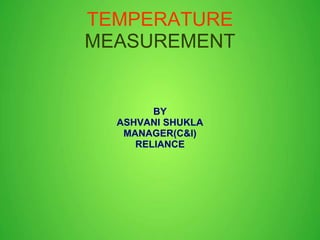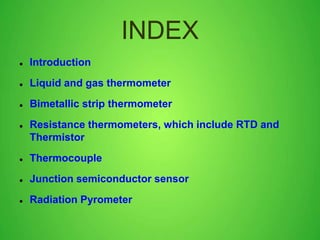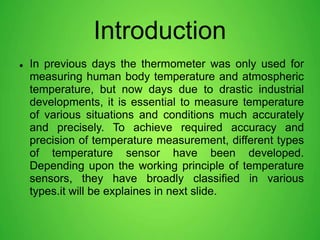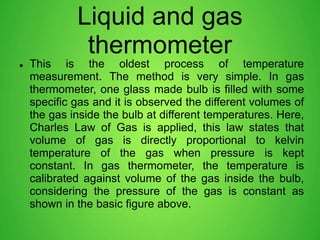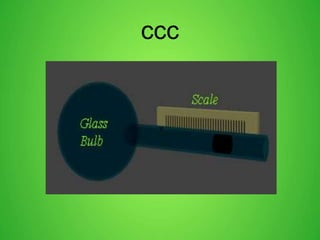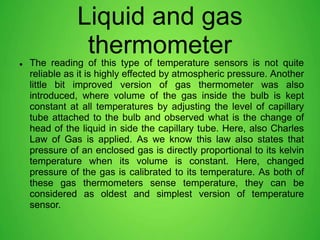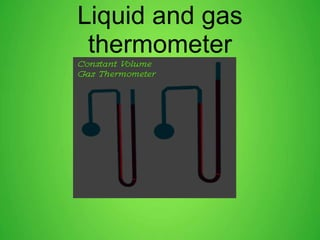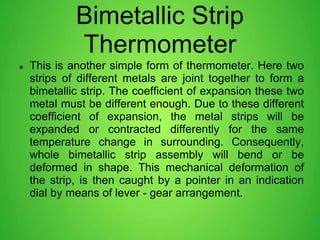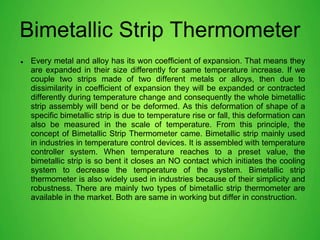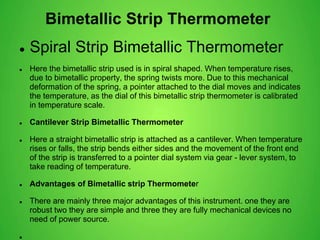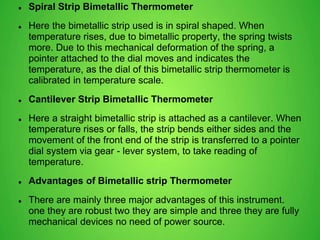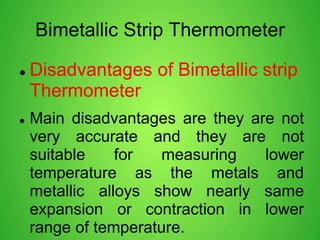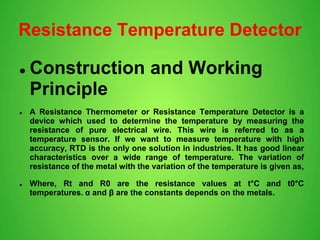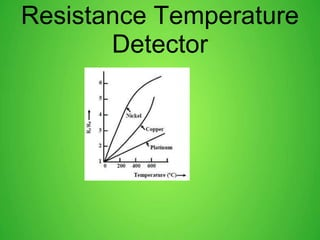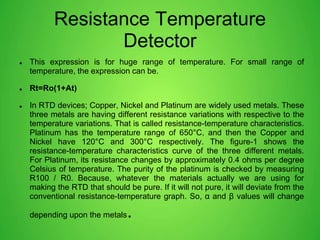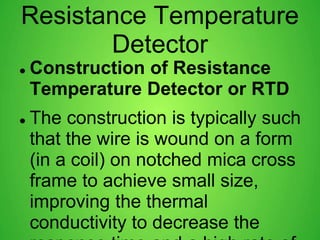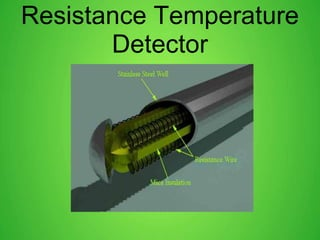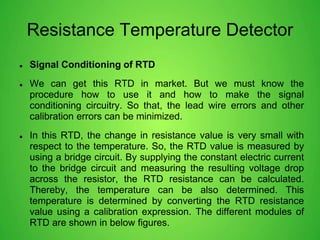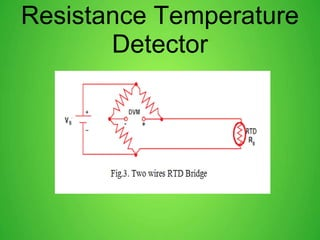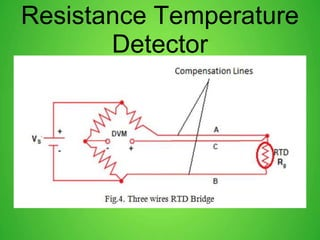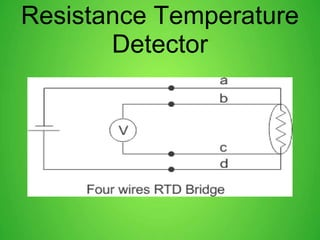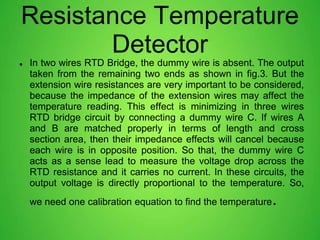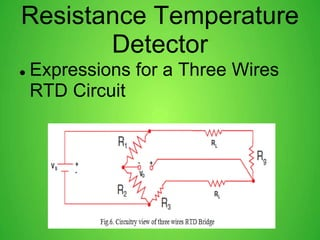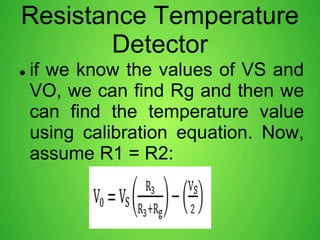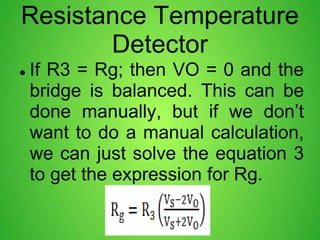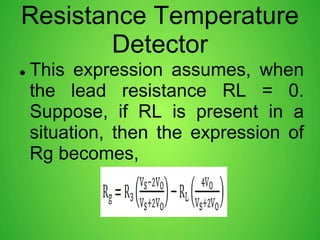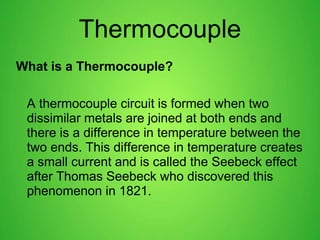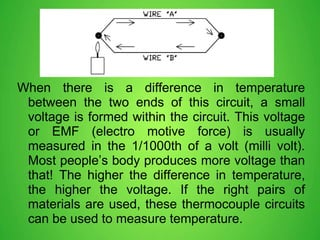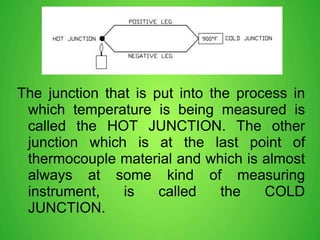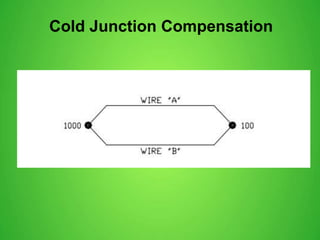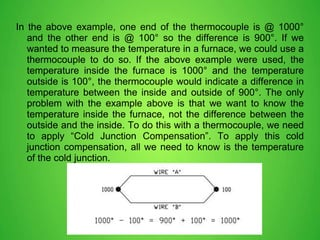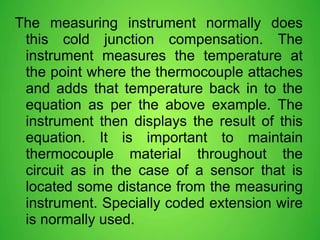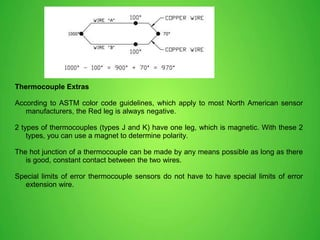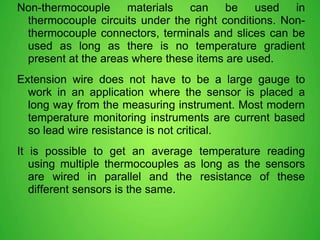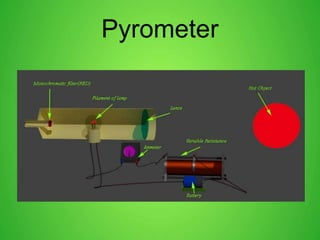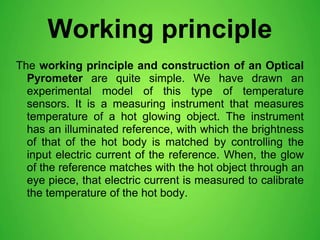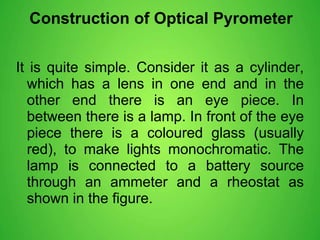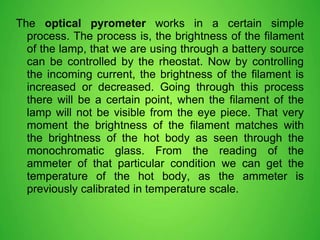1 von 39
Anzeige

### Temperature measurement

1. TEMPERATURE MEASUREMENT BY ASHVANI SHUKLA MANAGER(C&I) RELIANCE
2. INDEX  Introduction  Liquid and gas thermometer  Bimetallic strip thermometer  Resistance thermometers, which include RTD and Thermistor  Thermocouple  Junction semiconductor sensor  Radiation Pyrometer
3. Introduction  In previous days the thermometer was only used for measuring human body temperature and atmospheric temperature, but now days due to drastic industrial developments, it is essential to measure temperature of various situations and conditions much accurately and precisely. To achieve required accuracy and precision of temperature measurement, different types of temperature sensor have been developed. Depending upon the working principle of temperature sensors, they have broadly classified in various types.it will be explaines in next slide.
4. Liquid and gas thermometer  This is the oldest process of temperature measurement. The method is very simple. In gas thermometer, one glass made bulb is filled with some specific gas and it is observed the different volumes of the gas inside the bulb at different temperatures. Here, Charles Law of Gas is applied, this law states that volume of gas is directly proportional to kelvin temperature of the gas when pressure is kept constant. In gas thermometer, the temperature is calibrated against volume of the gas inside the bulb, considering the pressure of the gas is constant as shown in the basic figure above.
5. ccc v
6. Liquid and gas thermometer  The reading of this type of temperature sensors is not quite reliable as it is highly effected by atmospheric pressure. Another little bit improved version of gas thermometer was also introduced, where volume of the gas inside the bulb is kept constant at all temperatures by adjusting the level of capillary tube attached to the bulb and observed what is the change of head of the liquid in side the capillary tube. Here, also Charles Law of Gas is applied. As we know this law also states that pressure of an enclosed gas is directly proportional to its kelvin temperature when its volume is constant. Here, changed pressure of the gas is calibrated to its temperature. As both of these gas thermometers sense temperature, they can be considered as oldest and simplest version of temperature sensor.
7. Liquid and gas thermometer
8. Bimetallic Strip Thermometer  This is another simple form of thermometer. Here two strips of different metals are joint together to form a bimetallic strip. The coefficient of expansion these two metal must be different enough. Due to these different coefficient of expansion, the metal strips will be expanded or contracted differently for the same temperature change in surrounding. Consequently, whole bimetallic strip assembly will bend or be deformed in shape. This mechanical deformation of the strip, is then caught by a pointer in an indication dial by means of lever - gear arrangement.
9. Bimetallic Strip Thermometer  Every metal and alloy has its won coefficient of expansion. That means they are expanded in their size differently for same temperature increase. If we couple two strips made of two different metals or alloys, then due to dissimilarity in coefficient of expansion they will be expanded or contracted differently during temperature change and consequently the whole bimetallic strip assembly will bend or be deformed. As this deformation of shape of a specific bimetallic strip is due to temperature rise or fall, this deformation can also be measured in the scale of temperature. From this principle, the concept of Bimetallic Strip Thermometer came. Bimetallic strip mainly used in industries in temperature control devices. It is assembled with temperature controller system. When temperature reaches to a preset value, the bimetallic strip is so bent it closes an NO contact which initiates the cooling system to decrease the temperature of the system. Bimetallic strip thermometer is also widely used in industries because of their simplicity and robustness. There are mainly two types of bimetallic strip thermometer are available in the market. Both are same in working but differ in construction.
10. Bimetallic Strip Thermometer  Spiral Strip Bimetallic Thermometer  Here the bimetallic strip used is in spiral shaped. When temperature rises, due to bimetallic property, the spring twists more. Due to this mechanical deformation of the spring, a pointer attached to the dial moves and indicates the temperature, as the dial of this bimetallic strip thermometer is calibrated in temperature scale.  Cantilever Strip Bimetallic Thermometer  Here a straight bimetallic strip is attached as a cantilever. When temperature rises or falls, the strip bends either sides and the movement of the front end of the strip is transferred to a pointer dial system via gear - lever system, to take reading of temperature.  Advantages of Bimetallic strip Thermometer  There are mainly three major advantages of this instrument. one they are robust two they are simple and three they are fully mechanical devices no need of power source. 
11.  Spiral Strip Bimetallic Thermometer  Here the bimetallic strip used is in spiral shaped. When temperature rises, due to bimetallic property, the spring twists more. Due to this mechanical deformation of the spring, a pointer attached to the dial moves and indicates the temperature, as the dial of this bimetallic strip thermometer is calibrated in temperature scale.  Cantilever Strip Bimetallic Thermometer  Here a straight bimetallic strip is attached as a cantilever. When temperature rises or falls, the strip bends either sides and the movement of the front end of the strip is transferred to a pointer dial system via gear - lever system, to take reading of temperature.  Advantages of Bimetallic strip Thermometer  There are mainly three major advantages of this instrument. one they are robust two they are simple and three they are fully mechanical devices no need of power source.
12. Bimetallic Strip Thermometer  Disadvantages of Bimetallic strip Thermometer  Main disadvantages are they are not very accurate and they are not suitable for measuring lower temperature as the metals and metallic alloys show nearly same expansion or contraction in lower range of temperature.
13. Resistance Temperature Detector  Construction and Working Principle  A Resistance Thermometer or Resistance Temperature Detector is a device which used to determine the temperature by measuring the resistance of pure electrical wire. This wire is referred to as a temperature sensor. If we want to measure temperature with high accuracy, RTD is the only one solution in industries. It has good linear characteristics over a wide range of temperature. The variation of resistance of the metal with the variation of the temperature is given as,  Where, Rt and R0 are the resistance values at t°C and t0°C temperatures. α and β are the constants depends on the metals.
14. Resistance Temperature Detector
15. Resistance Temperature Detector  This expression is for huge range of temperature. For small range of temperature, the expression can be.  Rt=Ro(1+At)  In RTD devices; Copper, Nickel and Platinum are widely used metals. These three metals are having different resistance variations with respective to the temperature variations. That is called resistance-temperature characteristics. Platinum has the temperature range of 650°C, and then the Copper and Nickel have 120°C and 300°C respectively. The figure-1 shows the resistance-temperature characteristics curve of the three different metals. For Platinum, its resistance changes by approximately 0.4 ohms per degree Celsius of temperature. The purity of the platinum is checked by measuring R100 / R0. Because, whatever the materials actually we are using for making the RTD that should be pure. If it will not pure, it will deviate from the conventional resistance-temperature graph. So, α and β values will change depending upon the metals.
16. Resistance Temperature Detector  Construction of Resistance Temperature Detector or RTD  The construction is typically such that the wire is wound on a form (in a coil) on notched mica cross frame to achieve small size, improving the thermal conductivity to decrease the
17. Resistance Temperature Detector
18. Resistance Temperature Detector  Signal Conditioning of RTD  We can get this RTD in market. But we must know the procedure how to use it and how to make the signal conditioning circuitry. So that, the lead wire errors and other calibration errors can be minimized.  In this RTD, the change in resistance value is very small with respect to the temperature. So, the RTD value is measured by using a bridge circuit. By supplying the constant electric current to the bridge circuit and measuring the resulting voltage drop across the resistor, the RTD resistance can be calculated. Thereby, the temperature can be also determined. This temperature is determined by converting the RTD resistance value using a calibration expression. The different modules of RTD are shown in below figures.
19. Resistance Temperature Detector
20. Resistance Temperature Detector
21. Resistance Temperature Detector
22. Resistance Temperature Detector  In two wires RTD Bridge, the dummy wire is absent. The output taken from the remaining two ends as shown in fig.3. But the extension wire resistances are very important to be considered, because the impedance of the extension wires may affect the temperature reading. This effect is minimizing in three wires RTD bridge circuit by connecting a dummy wire C. If wires A and B are matched properly in terms of length and cross section area, then their impedance effects will cancel because each wire is in opposite position. So that, the dummy wire C acts as a sense lead to measure the voltage drop across the RTD resistance and it carries no current. In these circuits, the output voltage is directly proportional to the temperature. So, we need one calibration equation to find the temperature.
23. Resistance Temperature Detector  Expressions for a Three Wires RTD Circuit
24. Resistance Temperature Detector  if we know the values of VS and VO, we can find Rg and then we can find the temperature value using calibration equation. Now, assume R1 = R2:
25. Resistance Temperature Detector  If R3 = Rg; then VO = 0 and the bridge is balanced. This can be done manually, but if we don’t want to do a manual calculation, we can just solve the equation 3 to get the expression for Rg.
26. Resistance Temperature Detector  This expression assumes, when the lead resistance RL = 0. Suppose, if RL is present in a situation, then the expression of Rg becomes,
27. Thermocouple What is a Thermocouple? A thermocouple circuit is formed when two dissimilar metals are joined at both ends and there is a difference in temperature between the two ends. This difference in temperature creates a small current and is called the Seebeck effect after Thomas Seebeck who discovered this phenomenon in 1821.
28. When there is a difference in temperature between the two ends of this circuit, a small voltage is formed within the circuit. This voltage or EMF (electro motive force) is usually measured in the 1/1000th of a volt (milli volt). Most people’s body produces more voltage than that! The higher the difference in temperature, the higher the voltage. If the right pairs of materials are used, these thermocouple circuits can be used to measure temperature.
29. The junction that is put into the process in which temperature is being measured is called the HOT JUNCTION. The other junction which is at the last point of thermocouple material and which is almost always at some kind of measuring instrument, is called the COLD JUNCTION.
30. Cold Junction Compensation
31. In the above example, one end of the thermocouple is @ 1000° and the other end is @ 100° so the difference is 900°. If we wanted to measure the temperature in a furnace, we could use a thermocouple to do so. If the above example were used, the temperature inside the furnace is 1000° and the temperature outside is 100°, the thermocouple would indicate a difference in temperature between the inside and outside of 900°. The only problem with the example above is that we want to know the temperature inside the furnace, not the difference between the outside and the inside. To do this with a thermocouple, we need to apply “Cold Junction Compensation”. To apply this cold junction compensation, all we need to know is the temperature of the cold junction.
32. The measuring instrument normally does this cold junction compensation. The instrument measures the temperature at the point where the thermocouple attaches and adds that temperature back in to the equation as per the above example. The instrument then displays the result of this equation. It is important to maintain thermocouple material throughout the circuit as in the case of a sensor that is located some distance from the measuring instrument. Specially coded extension wire is normally used.
33. Thermocouple Extras According to ASTM color code guidelines, which apply to most North American sensor manufacturers, the Red leg is always negative. 2 types of thermocouples (types J and K) have one leg, which is magnetic. With these 2 types, you can use a magnet to determine polarity. The hot junction of a thermocouple can be made by any means possible as long as there is good, constant contact between the two wires. Special limits of error thermocouple sensors do not have to have special limits of error extension wire.
34. Non-thermocouple materials can be used in thermocouple circuits under the right conditions. Non- thermocouple connectors, terminals and slices can be used as long as there is no temperature gradient present at the areas where these items are used. Extension wire does not have to be a large gauge to work in an application where the sensor is placed a long way from the measuring instrument. Most modern temperature monitoring instruments are current based so lead wire resistance is not critical. It is possible to get an average temperature reading using multiple thermocouples as long as the sensors are wired in parallel and the resistance of these different sensors is the same.
35. Pyrometer
36. Working principle The working principle and construction of an Optical Pyrometer are quite simple. We have drawn an experimental model of this type of temperature sensors. It is a measuring instrument that measures temperature of a hot glowing object. The instrument has an illuminated reference, with which the brightness of that of the hot body is matched by controlling the input electric current of the reference. When, the glow of the reference matches with the hot object through an eye piece, that electric current is measured to calibrate the temperature of the hot body.
37. Construction of Optical Pyrometer It is quite simple. Consider it as a cylinder, which has a lens in one end and in the other end there is an eye piece. In between there is a lamp. In front of the eye piece there is a coloured glass (usually red), to make lights monochromatic. The lamp is connected to a battery source through an ammeter and a rheostat as shown in the figure.
38. The optical pyrometer works in a certain simple process. The process is, the brightness of the filament of the lamp, that we are using through a battery source can be controlled by the rheostat. Now by controlling the incoming current, the brightness of the filament is increased or decreased. Going through this process there will be a certain point, when the filament of the lamp will not be visible from the eye piece. That very moment the brightness of the filament matches with the brightness of the hot body as seen through the monochromatic glass. From the reading of the ammeter of that particular condition we can get the temperature of the hot body, as the ammeter is previously calibrated in temperature scale.
39. Thank You....................
Anzeige